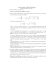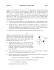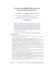# Homework #11

## Transcription

Homework #11
```OPTI 511R, Spring 2015
Problem Set 11
Prof. E. M. Wright
Due Thursday April 30, 2015
1. Consider a laser composed of a stable linear cavity with 2 mirrors each having power reflectivity
coefficients R = 0.98 and a homogeneously broadened (but no collisions) 10 cm long gain medium. The
gain medium is modeled as a 3-level system as diagramed below with population densities N1 , N2 , N3 .
NT = 1 × 1019 cm−3 is the total population density of this closed 3-level system. The spontaneous
decay rates between dipole allowed transitions (Γij ) are also shown. An external pumping rate P is
required for population inversion, where PT is the pumping rate required to reach the lasing threshold
on the 2-1 transition. The gain coefficient here for the 2-1 transition can be written as:
g=
∆N o · σ(ωo )
,
1 + I/Isat + 4(∆/Γ21 )2
where the resonant absorption cross section for this gain medium is σ = 3 × 10−20 cm2 .
(a) Argue why, based on the selection rules for dipole allowed transitions, there is no spontaneous
emission between levels 3-1 in this system.
(b) Write down the set of coupled rate equations for the 3-level system assuming P < PT .
(c) Assuming Γ32 >> P , derive the expression for the steady-state population inversion density
on the 2-1 transition, N2 − N1 , assuming P < PT .
(d) What is the minimum pumping rate needed to have gain rather than absorption of light resonant
with the 2-1 transition?
(e) Assume a shutter is momentarily placed inside the laser cavity to prevent it from lasing. If
P = 2Γ21 , make a detailed plot of the gain coefficient versus detuning for the 2-1 transition. Be sure
to indicate on the plot the numeric value of the gain coefficient at ∆ = 0 and the full-width at half
maximum in terms of parameters defined in this problem.
P N3 ! 32
N2 ! 21
N1 1
(f ) Assuming P = 2Γ21 and with shutter opened, is it possible for the laser to operate? Justify you
2.
(a) Suppose that the small-signal gain coefficient g0 (ω) of a Doppler-broadened gas laser has
a peak g0 (ω0 ) (at at the atomic resonance) that is twice the threshold gain coefficient gt . If g0 (ω) has
a FWHM of 3 GHz, about how many TEM0,0 axial modes could simultaneously lase if the cavity has
a length of 1 m?
(b) If this laser where mode-locked, what is the shortest possible pulse duration that could be achieved?
What is the repetition rate of the pulse train?
(c) What would the cavity length have to be (approximately) in order to make this laser operate
on only a single longitudinal mode?
(d) A CO2 laser has an unusually narrow Doppler-broadened transition linewidth of ∆ω ∼ 2π × (60
MHz) at FWHM. Approximately what cavity length would allow a CO2 laser to operate in a single
longitudinal mode?
3. (a) A ruby laser has mirror power reflectivity’s of R1 = 1 and R2 = 0.94, and a ruby gain rod
length of 5 cm (which is the same as the laser cavity length). The upper level lifetime is τ2 = 2×10−3 s.
Assuming a pumping level of g0 /gt =2, determine if this laser will exhibit relaxation oscillations by
(b) If this laser where Q-switched and achieved an extraction efficiency of η = 0.7, what would
be the approximate duration of the pulse?
2
```

### Nanocomp Pattern generators, PDF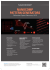### Laser Engraving - Source International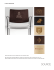### INVITATION TO: - Diowave Laser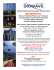### GASGLASS LASER NEW GAS FILL ANALYZER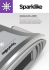### laser pointer pen sale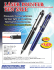### SPEcificAtionS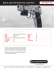### Here - Ultrazone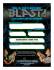### Panduit S100X150FAL datasheet: pdf### Legacy Sell Sheet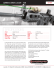### SerieS BL2000 - Baublys Laser GmbH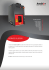### Laser Physics Homework 7, Due Date